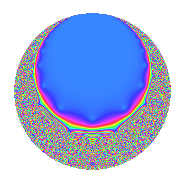# Properties

 Label 4.12.aLevel 4 Weight 12 Character orbit a Rep. character $$\chi_{4}(1,\cdot)$$ Character field $$\Q$$ Dimension 1 Newforms 1 Sturm bound 6 Trace bound 0

# Related objects

## Defining parameters

 Level: $$N$$ = $$4 = 2^{2}$$ Weight: $$k$$ = $$12$$ Character orbit: $$[\chi]$$ = 4.a (trivial) Character field: $$\Q$$ Newforms: $$1$$ Sturm bound: $$6$$ Trace bound: $$0$$

## Dimensions

The following table gives the dimensions of various subspaces of $$M_{12}(\Gamma_0(4))$$.

Total New Old
Modular forms 7 1 6
Cusp forms 4 1 3
Eisenstein series 3 0 3

The following table gives the dimensions of the cuspidal new subspaces with specified eigenvalues for the Atkin-Lehner operators and the Fricke involution.

$$2$$Dim.
$$-$$$$1$$

## Trace form

 $$q$$ $$\mathstrut -\mathstrut 516q^{3}$$ $$\mathstrut -\mathstrut 10530q^{5}$$ $$\mathstrut +\mathstrut 49304q^{7}$$ $$\mathstrut +\mathstrut 89109q^{9}$$ $$\mathstrut +\mathstrut O(q^{10})$$ $$q$$ $$\mathstrut -\mathstrut 516q^{3}$$ $$\mathstrut -\mathstrut 10530q^{5}$$ $$\mathstrut +\mathstrut 49304q^{7}$$ $$\mathstrut +\mathstrut 89109q^{9}$$ $$\mathstrut -\mathstrut 309420q^{11}$$ $$\mathstrut -\mathstrut 1723594q^{13}$$ $$\mathstrut +\mathstrut 5433480q^{15}$$ $$\mathstrut -\mathstrut 2279502q^{17}$$ $$\mathstrut +\mathstrut 4550444q^{19}$$ $$\mathstrut -\mathstrut 25440864q^{21}$$ $$\mathstrut -\mathstrut 7282872q^{23}$$ $$\mathstrut +\mathstrut 62052775q^{25}$$ $$\mathstrut +\mathstrut 45427608q^{27}$$ $$\mathstrut -\mathstrut 69040026q^{29}$$ $$\mathstrut -\mathstrut 141740704q^{31}$$ $$\mathstrut +\mathstrut 159660720q^{33}$$ $$\mathstrut -\mathstrut 519171120q^{35}$$ $$\mathstrut +\mathstrut 711366974q^{37}$$ $$\mathstrut +\mathstrut 889374504q^{39}$$ $$\mathstrut -\mathstrut 1225262214q^{41}$$ $$\mathstrut -\mathstrut 33606220q^{43}$$ $$\mathstrut -\mathstrut 938317770q^{45}$$ $$\mathstrut +\mathstrut 123214608q^{47}$$ $$\mathstrut +\mathstrut 453557673q^{49}$$ $$\mathstrut +\mathstrut 1176223032q^{51}$$ $$\mathstrut +\mathstrut 1106121582q^{53}$$ $$\mathstrut +\mathstrut 3258192600q^{55}$$ $$\mathstrut -\mathstrut 2348029104q^{57}$$ $$\mathstrut -\mathstrut 9062779932q^{59}$$ $$\mathstrut -\mathstrut 3854150458q^{61}$$ $$\mathstrut +\mathstrut 4393430136q^{63}$$ $$\mathstrut +\mathstrut 18149444820q^{65}$$ $$\mathstrut -\mathstrut 15313764676q^{67}$$ $$\mathstrut +\mathstrut 3757961952q^{69}$$ $$\mathstrut +\mathstrut 20619626328q^{71}$$ $$\mathstrut -\mathstrut 2063718694q^{73}$$ $$\mathstrut -\mathstrut 32019231900q^{75}$$ $$\mathstrut -\mathstrut 15255643680q^{77}$$ $$\mathstrut +\mathstrut 13689871472q^{79}$$ $$\mathstrut -\mathstrut 39226037751q^{81}$$ $$\mathstrut +\mathstrut 65570428908q^{83}$$ $$\mathstrut +\mathstrut 24003156060q^{85}$$ $$\mathstrut +\mathstrut 35624653416q^{87}$$ $$\mathstrut -\mathstrut 29715508854q^{89}$$ $$\mathstrut -\mathstrut 84980078576q^{91}$$ $$\mathstrut +\mathstrut 73138203264q^{93}$$ $$\mathstrut -\mathstrut 47916175320q^{95}$$ $$\mathstrut -\mathstrut 23439626206q^{97}$$ $$\mathstrut -\mathstrut 27572106780q^{99}$$ $$\mathstrut +\mathstrut O(q^{100})$$

## Decomposition of $$S_{12}^{\mathrm{new}}(\Gamma_0(4))$$ into irreducible Hecke orbits

Label Dim. $$A$$ Field CM Traces A-L signs $q$-expansion
$$a_2$$ $$a_3$$ $$a_5$$ $$a_7$$ 2
4.12.a.a $$1$$ $$3.073$$ $$\Q$$ None $$0$$ $$-516$$ $$-10530$$ $$49304$$ $$-$$ $$q-516q^{3}-10530q^{5}+49304q^{7}+\cdots$$

## Decomposition of $$S_{12}^{\mathrm{old}}(\Gamma_0(4))$$ into lower level spaces

$$S_{12}^{\mathrm{old}}(\Gamma_0(4)) \cong$$ $$S_{12}^{\mathrm{new}}(\Gamma_0(1))$$$$^{\oplus 3}$$